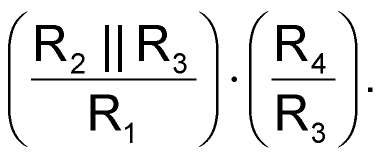# Two op amps provide averaged absolute value

## Texas Instruments TL082

The circuit in Figure 1 is useful when you need amplitude demodulation or an averaged absolute-value conversion. The circuit comprises two stages, the first of which, IC1A, is a differential-output absolute-value converter. The second stage, IC1B, is a traditional differential amplifier. The combination of the two stages performs single-ended absolute-value conversion but only if R3 >>R2. The C1 capacitors integrate the current flow and yield averaged voltages VA and VB. In addition, the capacitors ensure low ac-impedance points at nodes VA and VB when the output diodes are reverse-biased. The additional C2 capacitors in parallel with R4 resistors impart a second-order-lowpass-filter characteristic to the circuit and remove the remaining ac signal. From a practical point of view, you can choose R3 to be five to 10 times higher than R2.Figure 1. This single-ended, averaged absolute-value converter is useful for amplitude demodulation.

The gain of the circuit isIn most applications, you would choose the filter time constants τ1 =R2||R3 ·C1 and τ2 =R4 ·C2 to be equal. The circuit in Figure 1 is simple, symmetrical, and cost-effective. It also makes it easy to calculate and adjust the gain using one resistor, R1. Other advantages are that the circuit has equal delay for positive- and negative-going signals and that it doesn't need matched diodes.

## Materials on the topic

EDN